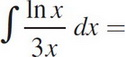# AP Calculus AB Practice Test 9

### Test Information8 questions24 minutes

Calculator Allowed

1. The average value of f(x) = e4x2 on the interval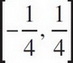is

2.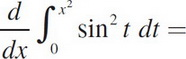3. Find the value(s) of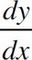of x2y + y2 = 5 at y = 1.

4. The graph of y = x3- 2x2 - 5x + 2 has a local maximum at

5. Approximate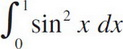using the Trapezoid Rule with n = 4, to three decimal places.

6. The volume generated by revolving about the x-axis the region above the curve y = x3, below the line y = 1, and between x = 0 and x = 1 is

7. A 20-foot ladder slides down a wall at 5 ft/sec. At what speed is the bottom sliding out when the top is 10 feet from the floor (in ft/sec)?

8.